Practice Test: Mechanical Engineering (ME)- 4

# Practice Test: Mechanical Engineering (ME)- 4 - Mechanical Engineering

Test Description

## 65 Questions MCQ Test GATE Mechanical (ME) 2024 Mock Test Series - Practice Test: Mechanical Engineering (ME)- 4

Practice Test: Mechanical Engineering (ME)- 4 for Mechanical Engineering 2023 is part of GATE Mechanical (ME) 2024 Mock Test Series preparation. The Practice Test: Mechanical Engineering (ME)- 4 questions and answers have been prepared according to the Mechanical Engineering exam syllabus.The Practice Test: Mechanical Engineering (ME)- 4 MCQs are made for Mechanical Engineering 2023 Exam. Find important definitions, questions, notes, meanings, examples, exercises, MCQs and online tests for Practice Test: Mechanical Engineering (ME)- 4 below.
Solutions of Practice Test: Mechanical Engineering (ME)- 4 questions in English are available as part of our GATE Mechanical (ME) 2024 Mock Test Series for Mechanical Engineering & Practice Test: Mechanical Engineering (ME)- 4 solutions in Hindi for GATE Mechanical (ME) 2024 Mock Test Series course. Download more important topics, notes, lectures and mock test series for Mechanical Engineering Exam by signing up for free. Attempt Practice Test: Mechanical Engineering (ME)- 4 | 65 questions in 180 minutes | Mock test for Mechanical Engineering preparation | Free important questions MCQ to study GATE Mechanical (ME) 2024 Mock Test Series for Mechanical Engineering Exam | Download free PDF with solutions
 1 Crore+ students have signed up on EduRev. Have you?
Practice Test: Mechanical Engineering (ME)- 4 - Question 1

### Choose the word or phrase which is nearest in meaning to the key word:             Pilfer

Practice Test: Mechanical Engineering (ME)- 4 - Question 2

### The following question comprises two words that have a certain relationship between them followed by four pairs of words. Select the pair that has same relationship as the original pair of words             Fox : Cunning

Practice Test: Mechanical Engineering (ME)- 4 - Question 3

### Replace the phrase printed in bold to make it grammatically correct? A twenty-first-century economy "cannot be held" hostage by power cuts nor travel on nineteenth-century roads.

Practice Test: Mechanical Engineering (ME)- 4 - Question 4

Improve the sentence with suitable options by replacing the underlined word.

He "lay" on the grass enjoying the sunshine.

Practice Test: Mechanical Engineering (ME)- 4 - Question 5
1. Fill in the blanks :

At times, we are all ______ to be mistaken.

Practice Test: Mechanical Engineering (ME)- 4 - Question 6

There are two solutions of wine and water, the concentration of wine being 0.4 and 0.6 respectively. If five liters of the first solution be mixed with fifteen liters of the second, find the concentration of wine in the resultant solution.

Detailed Solution for Practice Test: Mechanical Engineering (ME)- 4 - Question 6

Resultant concentration of wine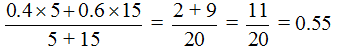Practice Test: Mechanical Engineering (ME)- 4 - Question 7

Rajiv bought some apples at the rate of 25 for Rs. 400 and an equal number at the rate of 30 for Rs. 270. He then sold all at the rate of 10 for Rs. 180. Find his profit/loss percentage

Detailed Solution for Practice Test: Mechanical Engineering (ME)- 4 - Question 7

Let Rajiv bought x apples of each kind.

Total cost price of Rajiv =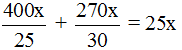Total selling price of Rajiv =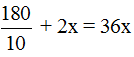SP > CP , Hence he makes profit;

Profit percentage  =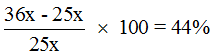Practice Test: Mechanical Engineering (ME)- 4 - Question 8

The probability of a lottery ticket being a prized ticket is 0.2. When 4 tickets are purchased, the probability of winning a prize on atleast one ticket is -.

Practice Test: Mechanical Engineering (ME)- 4 - Question 9

2 men and 3 boys can do a piece of work in 10 days, while 3 men and 2 boys can do the same work in 8 days. In how many days can 2 men and 1 boy do the work ?

Detailed Solution for Practice Test: Mechanical Engineering (ME)- 4 - Question 9

Let work done by 1 man in 1 day = x units

Let work done by 1 boy in 1 day = y units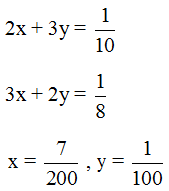2 men and 1 boy can finish work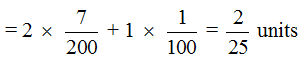No. of days taken by 2 men and 1 boy = = 12.5 days

Practice Test: Mechanical Engineering (ME)- 4 - Question 10

In an exam, 60% of the candidates passed in Maths and 70% candidates passed in English and 10% of the candidates failed in both the subjects, 300 candidates passed in both the subjects. Find the total number of candidates appeared in the exam, if they took test in only two subjects that is Math and English.

Detailed Solution for Practice Test: Mechanical Engineering (ME)- 4 - Question 10

Let total number of students appear be X.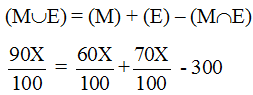X = 750

Practice Test: Mechanical Engineering (ME)- 4 - Question 11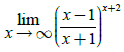is equal to

Detailed Solution for Practice Test: Mechanical Engineering (ME)- 4 - Question 11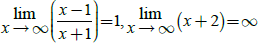Thus 1 in determinant form occurs adding and subtracting 1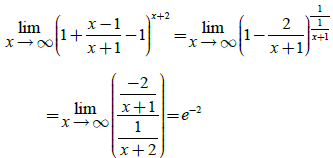Practice Test: Mechanical Engineering (ME)- 4 - Question 12

A fair coin is tossed repeatedly. The probability of getting a result in the fifth toss different from those obtained in the first four tosses.

Detailed Solution for Practice Test: Mechanical Engineering (ME)- 4 - Question 12

Let  E1   be the event that head occurs in fifth toss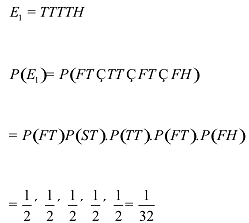E2   be the event that first four heads and fifth tail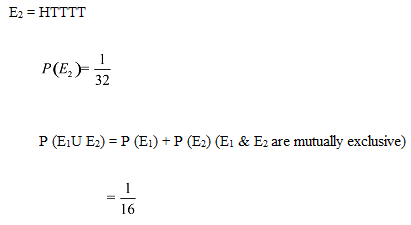Practice Test: Mechanical Engineering (ME)- 4 - Question 13

Other Roots of the equationif one root is same as one root of x2 + 10x + 9 = 0

Detailed Solution for Practice Test: Mechanical Engineering (ME)- 4 - Question 13

Given determinant on expansion reduces to the equation
?    x3 – 67x+ 126 = 0  -------------(1)
?    Given equation x2+10x+ 9 has roots -9, -1
?    {– 9 is also a root of (1)}
?        So L.H.S of (1) is exactly divisible by x 9. On dividing x3 – 67x+ 126  by  x+ 9 we get quotient  x2 – 9x+ 14  Roots of x2– 9x +14 which are 2 and 7 are also roots of (1)

Practice Test: Mechanical Engineering (ME)- 4 - Question 14

A speaks truth in 60% cases and B speaks truth in 70% cases. The probability that they will say the same thing while describing a single event is

Detailed Solution for Practice Test: Mechanical Engineering (ME)- 4 - Question 14

P (A) = Probability that A speaks truth = 0.6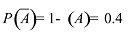P(B) = Probability that B speaks the truth = 0.7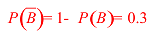E1 : Both speaks truth
E2 : Both speak false
E : Both speaks the same thing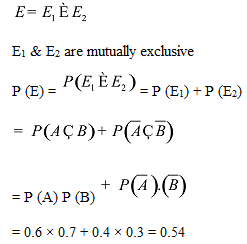Practice Test: Mechanical Engineering (ME)- 4 - Question 15

While water passes through a given pipe at mean velocity V1. The flow is found to change from Laminar to turbulent. If another fluid of specific gravity 0.8 and coefficient of viscosity 20% of that of water, is passed through the same pipe, the transition of flow from Laminar to turbulent is expected if the flow velocity is

Detailed Solution for Practice Test: Mechanical Engineering (ME)- 4 - Question 15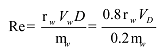Practice Test: Mechanical Engineering (ME)- 4 - Question 16

A cylinder contains 0.12 m3 of air at 1 bar and 900c  . It is compressed to 0.03 m3, the final pressure being 6 bar. The index of compression will be

Detailed Solution for Practice Test: Mechanical Engineering (ME)- 4 - Question 16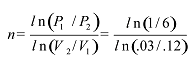n = 1.292

Practice Test: Mechanical Engineering (ME)- 4 - Question 17

The rpm of steel bar of 150 mm diameter which is turned at 50 m/min speed with carbide tool is.

Detailed Solution for Practice Test: Mechanical Engineering (ME)- 4 - Question 17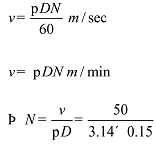N = 106.1 rpm

Practice Test: Mechanical Engineering (ME)- 4 - Question 18

A nuclear fuel element is in the form of a solid sphere of 12.5 mm radius. It’s thermal conductivity is 24.2 W/mK. The heat generation rate is 50 × 106 W/m3. The heat generated is     absorbed by a fluid at 2000C . If the maximum temperature is limited to 3600C , the convection coefficient (W/m2K) required is

Detailed Solution for Practice Test: Mechanical Engineering (ME)- 4 - Question 18

Amount of heat generated = Heat convected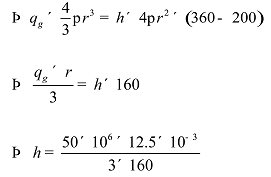h = 1302.08 watt / m2 k

Practice Test: Mechanical Engineering (ME)- 4 - Question 19

Consider the PERT network shown  below: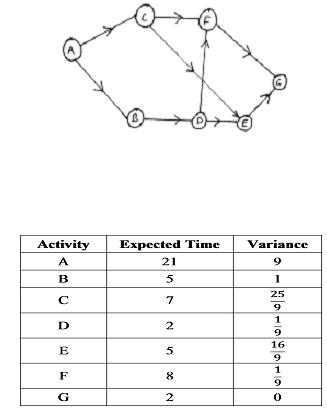The desired completion date for the project if probability of finishing the project is 19% (Z(0.87) = 0.19) is.

Detailed Solution for Practice Test: Mechanical Engineering (ME)- 4 - Question 19

Critical path
A - B - D - F - G
Project time = 38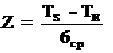TS = 35 Weeks

Practice Test: Mechanical Engineering (ME)- 4 - Question 20

In rolling process, roll diameter is 800 mm. The thickness of 60 mm strip is to be reduced to 40 mm in single pass. Coefficient of friction is

Detailed Solution for Practice Test: Mechanical Engineering (ME)- 4 - Question 20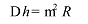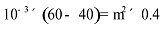m = 0.223

Practice Test: Mechanical Engineering (ME)- 4 - Question 21

A gas expands from an initial state where the pressure is 340 kPa and volume is 0.0425 m3 to a final pressure of 136 kPa. If the gas follows PV2 = c, then the work for the process (in kJ) will be.

Detailed Solution for Practice Test: Mechanical Engineering (ME)- 4 - Question 21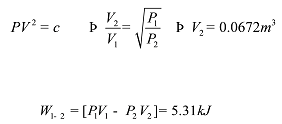Practice Test: Mechanical Engineering (ME)- 4 - Question 22

An item is produced at the rate of 50 items per day. The demand occurs at the rate of 25 items per day. If the setup cost is Rs. 100 per setup and holding cost is Rs 0.01 per unit of item per day. The economic lot size is:

Detailed Solution for Practice Test: Mechanical Engineering (ME)- 4 - Question 22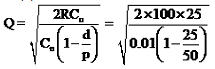= 1000 items

Practice Test: Mechanical Engineering (ME)- 4 - Question 23

In a pipe flow, the head loss due to friction is 6m. If the power transmitted through the pipe has to be maximum then the total head at the inlet will have to be maintained as.

Detailed Solution for Practice Test: Mechanical Engineering (ME)- 4 - Question 23

Head lost due to friction is 6 m. Power transmitted is maximum when the friction head is 1/3rd of the supply head supply head is 18 m

Practice Test: Mechanical Engineering (ME)- 4 - Question 24

In a cutting operation, angle of approach is 750  and feed is 0.32 mm/rev. The value of underformed chip thickness is.

Practice Test: Mechanical Engineering (ME)- 4 - Question 25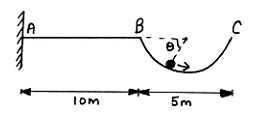Portion BC is semi-circular . If after several oscillation ball of mass 10 kg comes to rest. The bending moment at A when ball is at rest will be ? Take g = 9.81 m/s2

Detailed Solution for Practice Test: Mechanical Engineering (ME)- 4 - Question 25

At rest, ball is at lower most potion of semi circle
BM at A = l0g × (10 2.5)
= 10 × 9.81 × 12.5
= 1226.25 N-m

Practice Test: Mechanical Engineering (ME)- 4 - Question 26

An orthogonal cutting operation is being carried out under following conditions. Cutting speed = 4m/s, depth of cut = 0.5 mm,Chip thickness = 0.6 mm,Chip velocity is:-

Detailed Solution for Practice Test: Mechanical Engineering (ME)- 4 - Question 26

Chip velocity, VC = V × r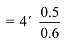= 3.33 m/s

Practice Test: Mechanical Engineering (ME)- 4 - Question 27

A two stage compressor takes in air at 1.1 bars and discharges at 20 bars. For max efficiency, the intermediate pressure is.

Detailed Solution for Practice Test: Mechanical Engineering (ME)- 4 - Question 27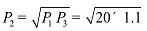P2 = 4.7 bar

Practice Test: Mechanical Engineering (ME)- 4 - Question 28

In case of Partial bearing, the angle of contact between the bush and journal is

Practice Test: Mechanical Engineering (ME)- 4 - Question 29

In a tool life test, doubling the cutting speed reduces the tool life to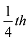of the original. Taylor’s tool life index is.

Detailed Solution for Practice Test: Mechanical Engineering (ME)- 4 - Question 29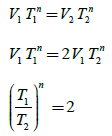(4)n = 2

n = 0.5

Practice Test: Mechanical Engineering (ME)- 4 - Question 30

A fluid jet is discharging from a 100 mm nozzle and the vena contracta formed has a diameter of 90 mm. If the coefficient of velocity is 0.95, then the coefficient of discharge for the nozzle is.

Detailed Solution for Practice Test: Mechanical Engineering (ME)- 4 - Question 30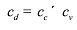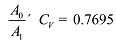Practice Test: Mechanical Engineering (ME)- 4 - Question 31

The purpose of helical grooves in a twist drill is to
1.    Improve the stiffness
2.    Save  tool material
3.    Provide space for chip removal
4.    Provide rake angle for cutting edge
Select the correct choice given below

Practice Test: Mechanical Engineering (ME)- 4 - Question 32

A  heat engine receives heat from a source  at 1200 K at a rate of 500 kW and rejects waste heat to a medium at 300 K. The power output of heat engine is 180 kW. The irreversibility rate associated is.

Detailed Solution for Practice Test: Mechanical Engineering (ME)- 4 - Question 32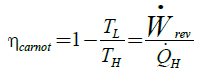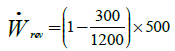Irreversibility Rate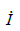= Reversible power – useful Power

375 – 180
= 195 kW

Practice Test: Mechanical Engineering (ME)- 4 - Question 33

Consider the following statements about the v engine, when the angle between the cylinder center lines is 900 .

1.    The resultant unbalanced primary force is m r w2
2.    Primary unbalance force can be completely balanced by a mass placed at a suitable radius diametrically opposite to the crank
3.    Secondary unbalanced force along the engine centre line is zero
4.    Resultant secondary unbalance force is zero.
Which of the above statements is/are incorrect

Practice Test: Mechanical Engineering (ME)- 4 - Question 34

When the annual demand of a product is 2400 units, the EOQ is 2000 units. If the annual demand is 4800 units, the EOQ will be:

Detailed Solution for Practice Test: Mechanical Engineering (ME)- 4 - Question 34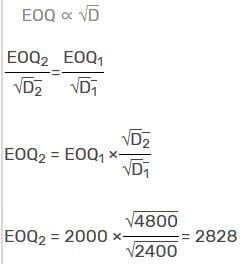Practice Test: Mechanical Engineering (ME)- 4 - Question 35

A cantilever beam AB shown in the figure has an extension BCD attached to the free end. A force P acts at the end of the extension. What is the ratio of a/L, so that vertical deflection at point B is zero?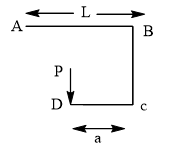Detailed Solution for Practice Test: Mechanical Engineering (ME)- 4 - Question 35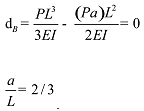Practice Test: Mechanical Engineering (ME)- 4 - Question 36

For an analytic function f (x iy) = u (x, y) iv (x, y), u is given by u = 4xy+ x. The expression for V considering K to  be a constant

Detailed Solution for Practice Test: Mechanical Engineering (ME)- 4 - Question 36

Since f is analytic, it will satisfy Cauchy. Riemann equations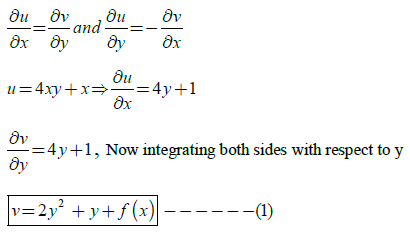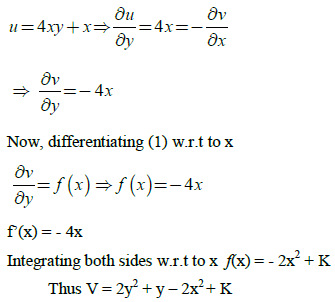Practice Test: Mechanical Engineering (ME)- 4 - Question 37

Consider the differential equation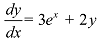with initial condition y (o) = 0. Taking step size of 0.1 the value of y at x = 0.1. Using fourth order Runge – kutta method

Detailed Solution for Practice Test: Mechanical Engineering (ME)- 4 - Question 37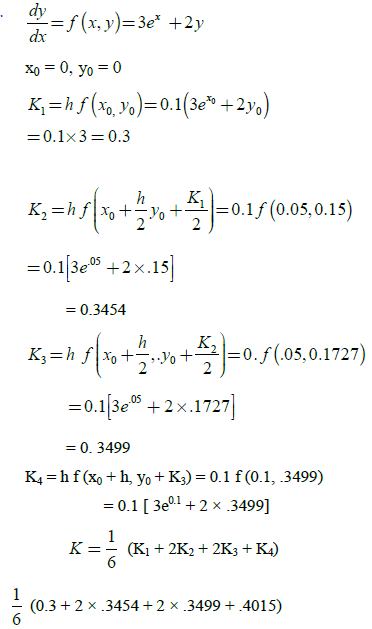= .34868

Practice Test: Mechanical Engineering (ME)- 4 - Question 38

Which condition of Rankine cycle has highest efficiency when operating between same pressure limits?

Detailed Solution for Practice Test: Mechanical Engineering (ME)- 4 - Question 38

The efficiency of ideal regenerative cycle is exactly equal to the corresponding Carnot cycle. Hence it is maximum.

Practice Test: Mechanical Engineering (ME)- 4 - Question 39

The inverse Laplace transform of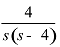Detailed Solution for Practice Test: Mechanical Engineering (ME)- 4 - Question 39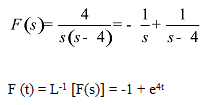Practice Test: Mechanical Engineering (ME)- 4 - Question 40

Find the natural frequency of vibration of the system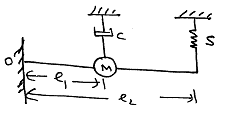Detailed Solution for Practice Test: Mechanical Engineering (ME)- 4 - Question 40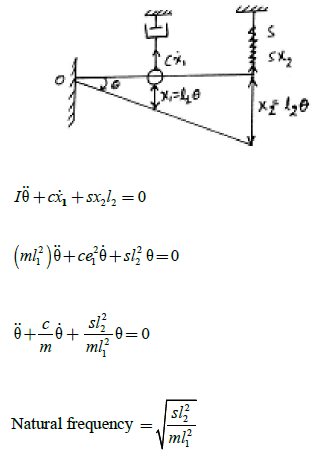Practice Test: Mechanical Engineering (ME)- 4 - Question 41

In Bell-Coleman refrigeration plant, air is drawn from cold chamber at pressure of  2 bar and 100C  , then compressed to 10 bar and then cooled to 250C in cooler before expansion in exparder. Refrigeration effect per kg of air is ( g = 1.4, CP = 1.09 kJ/kg K)

Detailed Solution for Practice Test: Mechanical Engineering (ME)- 4 - Question 41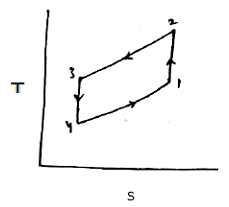T1 = 283 K,             T3 = 298 K

= 447.7 K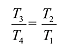T4  = 188.3
R.E./kg = CP (T1 – T4)
= 95.55 kJ

Practice Test: Mechanical Engineering (ME)- 4 - Question 42

A closed tank containing water is partly filled with water and the air space above it is under pressure. A 5 c.m. hose connected to the tank discharges water to atmosphere on to the roof of a building 3 m above the level of water in the tank, if friction losses are 1.5 m of water, what air pressure must be maintained in the tank to deliver 15 L/s to the roof?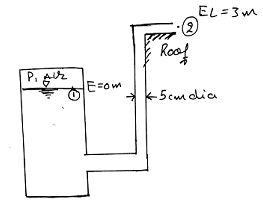Detailed Solution for Practice Test: Mechanical Engineering (ME)- 4 - Question 42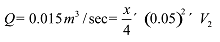V2 = 7.639 m/s
By applying Bernoulli equation to point 1and  2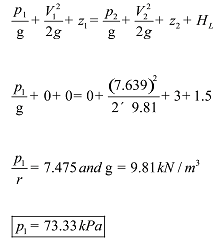Practice Test: Mechanical Engineering (ME)- 4 - Question 43

Calculate the elongation of copper bar of solid circular cross-section with tapered ends when it is stretched by axial loads of magnitude of 30 kN.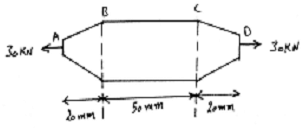Diameter at cross section  A, B, C and D are 0.5, 1, 1 and 0.5 cm respectively. Take E = 120 GPa

Detailed Solution for Practice Test: Mechanical Engineering (ME)- 4 - Question 43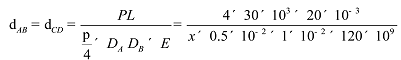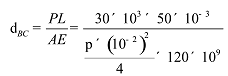= 1.59 × 10-4 m
Total elongation = (2 × 0.12732 0.15915) mn
= 0.4138 mm

Practice Test: Mechanical Engineering (ME)- 4 - Question 44

Cylindrical  tank of 1.0 m diameter and 5 m total length has hemispherical ends. It contains liquid oxygen which has boiling point and heat of vapourisation -1800C  and 210 kJ/kg respectively. It is required to insulate the tank so as to reduce the boit off rate of oxygen is steady state to 14 kg/h. Assume room temperature outside the insulation as 250C. If the maximum thickness of the insulating material is limited to 70 mm, the thermal conductivity(W/m0C) is

Detailed Solution for Practice Test: Mechanical Engineering (ME)- 4 - Question 44

r1 = 0.5 m
r2 = 0.57 m
ti = - 180°C, t0 = 25°C
Boil off rate = 14 kg/hr

hfg = 210 kJ/kg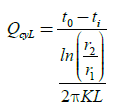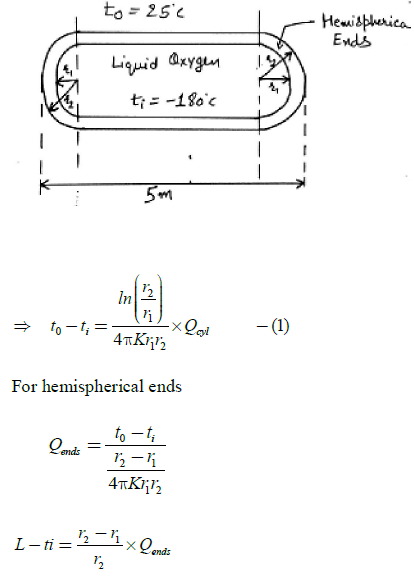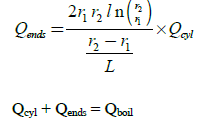= 14 × 210 = 2940
Also L + 2r1 = 5
L = 4 m
Qcyl = 644.69 watt
So, K = 0.0164 W /m0C

Practice Test: Mechanical Engineering (ME)- 4 - Question 45

Brass billet is to be extruded from its initial diameter of 40 mm to final diameter of 20 mm. The extrusion constant is 200 MPa. Force required for extrusion is

Detailed Solution for Practice Test: Mechanical Engineering (ME)- 4 - Question 45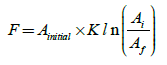= 348237 N
= 0.348 MN

Practice Test: Mechanical Engineering (ME)- 4 - Question 46

An ideal reheat Rankine cycle operates between the pressure limits of 10 kPa and 8 MPa, with eheat occurring at 4 MPa. The temperature of steam at the inlet of both turbines is 5000 C, and the enthalpy of steam is 3185 kJ/kg at the exit of the high pressure turbine, and 2247 kJ/kg at the exit of the low pressure turbine. Disregarding the pump work, the efficiency of the cycle is.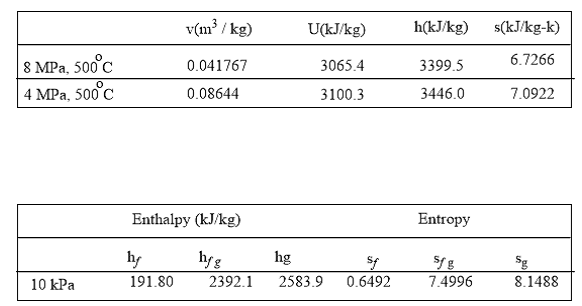Detailed Solution for Practice Test: Mechanical Engineering (ME)- 4 - Question 46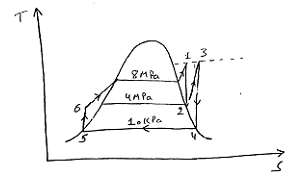h1 = 339.5 kJ/kg
h3 = 3446 kJ/kg
h2 = 3185 kJ/kg3
h4 = 2247 kJ/kg
h5 = 191.81 = h6
wnet = wT – wP [ wP = 0]
= (3399.5 – 3185) (3446 – 2247)
= 1413.5 kJ/kg = WT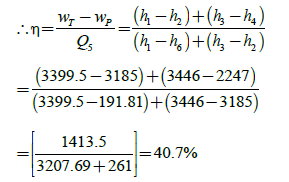Practice Test: Mechanical Engineering (ME)- 4 - Question 47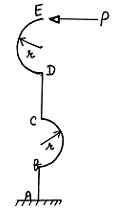The structure is carrying a horizontal load P at E. Legs AB and CD are vertical. The stress resultants in AB segment are

Practice Test: Mechanical Engineering (ME)- 4 - Question 48

Find entropy change of air in a polytropic equation where pressure is doubled & volume becomes one third. (Cp = 1 kJ/kgK, CV= 0.7 kJ/kgK)

Detailed Solution for Practice Test: Mechanical Engineering (ME)- 4 - Question 48

Tds =  CVdT PdV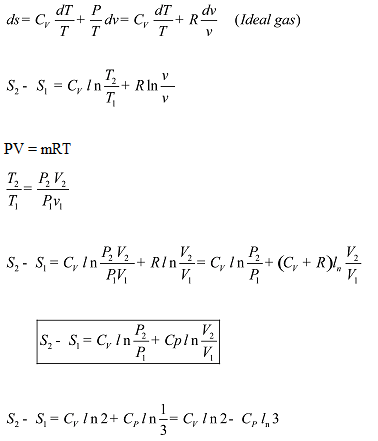= - 0.6134 kJ/kg

Practice Test: Mechanical Engineering (ME)- 4 - Question 49

Number of chlorine atoms in R – 12 are:-

Detailed Solution for Practice Test: Mechanical Engineering (ME)- 4 - Question 49

R – 012
R (m – 1) (n 1) p
m = 1, n = 0, p = 2
2m 2 = p q n
2(1) 2 = 2 q 0
q = 2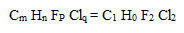So, two chlorine atoms

Practice Test: Mechanical Engineering (ME)- 4 - Question 50

200 kW is transmitted by a single reduction gear. The pitch circle diameter of pinion is 200 mm and its speed 750 rpm. The teeth are of involute profile with pressure angle equal to 200 .
The radial force acting on each wheel when transmitting the given power

Detailed Solution for Practice Test: Mechanical Engineering (ME)- 4 - Question 50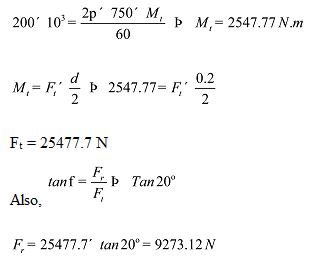Practice Test: Mechanical Engineering (ME)- 4 - Question 51

In a engine working on otto cycle the temp. at the beginning and end of the compression are 500C and 3730C . The compression ratio and air standard efficiency of the engine is.

Detailed Solution for Practice Test: Mechanical Engineering (ME)- 4 - Question 51

Compression ratio,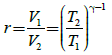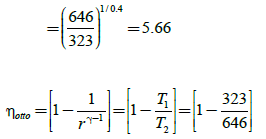= 0.5 = 50 %

Practice Test: Mechanical Engineering (ME)- 4 - Question 52

In the production shop of a company the breakdown of the machine is found to follow poisson distribution with an average rate of 3 machines per hour. Break down time at one machine
costs 40 rupees per hour to the company. The company hires a repairman who demands rupees 20 per hour and will repair the broken down machines following the exponential distribution at the rate of 4 per hour. The total cost involved in hiring the repairman is

Detailed Solution for Practice Test: Mechanical Engineering (ME)- 4 - Question 52

λ = 3/hr

μ = 4/hr

Total waiting time in system for one machine is

=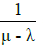= 1 hour

The down time of 3 machines is
= 3 × 1 = 3 hour
Down time cost = 40 × 3 = Rs 120
Repairman cost = 3 × 20 = Rs 60
Total cost = 120 60 = Rs 180

Practice Test: Mechanical Engineering (ME)- 4 - Question 53

Figure shows the linear power characteristics, voltage and arc length is related to each other by a linear equation V = 20 + 2l. What is the value of current when arc length is 5 mm (l is in
mm).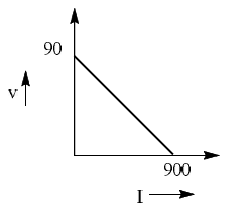Detailed Solution for Practice Test: Mechanical Engineering (ME)- 4 - Question 53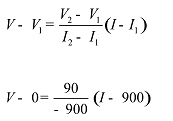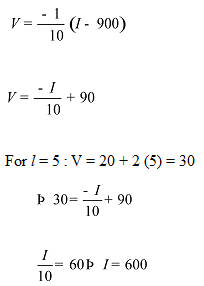Practice Test: Mechanical Engineering (ME)- 4 - Question 54

If all the dimensions of a vertically subtended circular bar are doubled, then the maximum stress produced in it due to its own weight will

Detailed Solution for Practice Test: Mechanical Engineering (ME)- 4 - Question 54

Max. stress occurs at point where full weight acts.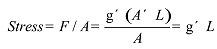Finally, stress = r × 2 L
Stress will be doubled

Practice Test: Mechanical Engineering (ME)- 4 - Question 55

Particle A makes a perfectly elastic collision with another particle B at rest. They fly apart in opposite direction with equal speeds. The ratio of their masses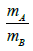is

Detailed Solution for Practice Test: Mechanical Engineering (ME)- 4 - Question 55

m1 = mA, m2 = mB, u1 = u, u2 = 0, v1 =v, v2 = - v
By principle of conservation of linear momentum.
m1 u 0 = m1 V m2 (-v) = (m1 – m2) v
By principle of conservation of K.E.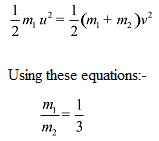Practice Test: Mechanical Engineering (ME)- 4 - Question 56

A hole with 40 mm diameter and 50 mm depth is to be drilled in mild steel component. The cutting speed can be taken as 65 m/min and feed rate is 0.25 mm/rev. The time required to drill
the hole in minutes is [Take drill point angle1180 and over travel 3mm]

Detailed Solution for Practice Test: Mechanical Engineering (ME)- 4 - Question 56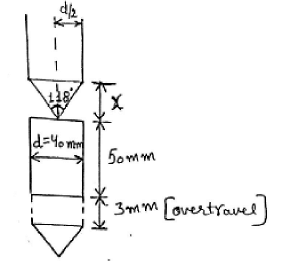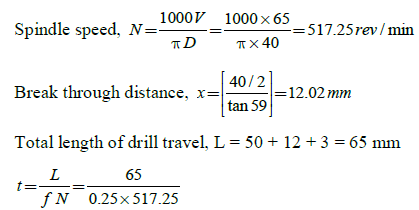T = 0.5026 min

Practice Test: Mechanical Engineering (ME)- 4 - Question 57

A flat steel bar with constant EI and length L it bent by couples applied at the ends so that the mid point deflection is δ(δ<<L). Then the value of applied couple M is?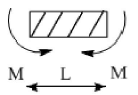Detailed Solution for Practice Test: Mechanical Engineering (ME)- 4 - Question 57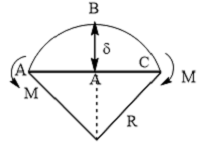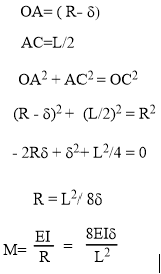Practice Test: Mechanical Engineering (ME)- 4 - Question 58

The variation of saturation pressure with saturation temperature for a liquid is 0.1 bar/k. The specific volume of saturated vapour and dry saturated liquid at 400 K are 251m3/kg and 0.001 m3/kg . The latent heat of vaporization using clausius clapeyron equation is.

Detailed Solution for Practice Test: Mechanical Engineering (ME)- 4 - Question 58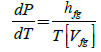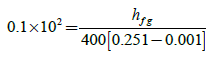hfg =  1000kJ / kg

Practice Test: Mechanical Engineering (ME)- 4 - Question 59

A steel washer is of 46 mm outer diameter and 24 mm inner hole diameter and is 4 mm thick.Maximum shear stress is 40.5 N/mm2 and percentage reduction is 22.Work done (in Joules) will be

Detailed Solution for Practice Test: Mechanical Engineering (ME)- 4 - Question 59

Production of washer includes blanking of outer diameter and piercing of inner  hole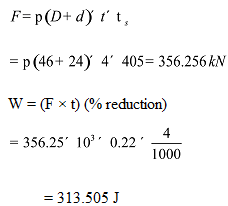Practice Test: Mechanical Engineering (ME)- 4 - Question 60

In a PERT network the expected time to finish one activity is 22 days and variance of the activity is 16 days. The ratio of pessimistic time to optimistic time if most likely time for finishing the activity is 23 days is

Detailed Solution for Practice Test: Mechanical Engineering (ME)- 4 - Question 60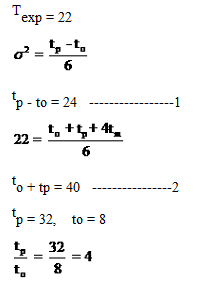Practice Test: Mechanical Engineering (ME)- 4 - Question 61

In beam ABCD, there is a roller support at B abd C as shown in the figure. If 200 kN load is applied at the indicant of BC. The shear force at the support A will be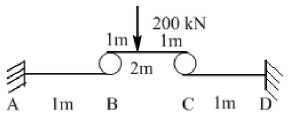Detailed Solution for Practice Test: Mechanical Engineering (ME)- 4 - Question 61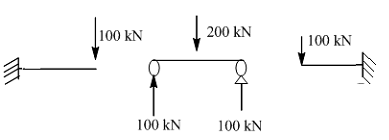SF at A = 100 kN

Practice Test: Mechanical Engineering (ME)- 4 - Question 62

Which one of the following is not the correct statement about control volume?

Practice Test: Mechanical Engineering (ME)- 4 - Question 63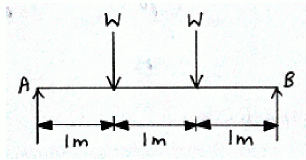Transverse natural frequency if single load is applied is 100 Hz. Find the frequency if both loads are applied simultaneously

Detailed Solution for Practice Test: Mechanical Engineering (ME)- 4 - Question 63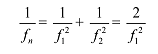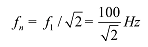Practice Test: Mechanical Engineering (ME)- 4 - Question 64

4800 kJ of heat is being transferred from constant temperature source at 800 K to another at 600 K when environment temperature is 200 K. The loss of available energy is.

Detailed Solution for Practice Test: Mechanical Engineering (ME)- 4 - Question 64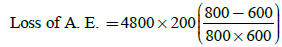= 2 × 200
= 400 kJ

Practice Test: Mechanical Engineering (ME)- 4 - Question 65

In a certain double pipe heat exchanger hot water flow at a rate of 5000 kg/h. and gets cooled from 950C to 650C . At the same time,50000 kg/h of cooling water at 300C enters the heat exchanger. The flow conditions, are such that overall heat transfer coefficient remains constant at 2270  W/m2K. Assume two streams are in parallel flow and for both the streams Cp = 4.2 kJ/kg K. The effective heat transfer area is

## GATE Mechanical (ME) 2024 Mock Test Series

27 docs|243 tests
Information about Practice Test: Mechanical Engineering (ME)- 4 Page
In this test you can find the Exam questions for Practice Test: Mechanical Engineering (ME)- 4 solved & explained in the simplest way possible. Besides giving Questions and answers for Practice Test: Mechanical Engineering (ME)- 4, EduRev gives you an ample number of Online tests for practice

## GATE Mechanical (ME) 2024 Mock Test Series

27 docs|243 tests